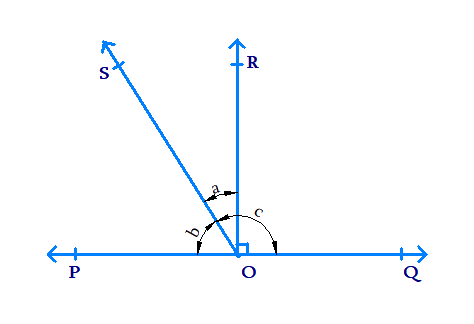# Ex.6.1 Q5 Lines and Angles Solution - NCERT Maths Class 9

Go back to  'Ex.6.1'

## Question

In the given figure, $$POQ$$ is a line. Ray $$OR$$ is perpendicular to line $$PQ.\;OS$$ another ray lying between rays $$OP$$ and $$OR.$$ Prove that \begin{align} \angle ROS =\frac {1}{2}(\angle QOS - \angle POS).\end{align}Video Solution
Lines And Angles
Ex 6.1 | Question 5

## Text Solution

What is known?

$$OR$$ is perpendicular to $$PQ.$$ $$\angle ROQ = \angle ROP =90^ {\circ}.$$

What is unknown?

To prove that: \begin{align} \angle ROS =\frac {1}{2}(\angle QOS - \angle POS)\end{align}

Reasoning:

When a ray intersects a line, then the sum of adjacent angles so formed is $$180^ {\circ}.$$

Steps:

Let  $$\angle ROS = a, \angle POS = b$$  and $$\angle SOQ = c.$$

To prove that: \begin{align}a= \frac{1}{2}(c-b).\end{align}

Since $$\angle ROQ = \angle ROP = 90 ^ { \circ }$$

We can say,

\begin{align} \angle POS + \angle SOR &= \angle POR \end{align}

\begin{align}\rm{} b + a &= 90 ^ { \circ } \ldots \ldots \ldots ( 1 ) \end{align}

Line $$PQ$$ is intersected by ray $$OS.$$

Hence, \begin{align}\angle POS + \angle SOQ = \,& b + c = 180 ^ { \circ } \end{align}

\begin{align}& b + c = 180 ^ { \circ } \ldots \ldots \ldots (2) \end{align}

From equation (1), we get: $$a + b = 90^ {\circ}$$

Multiplying by $$2$$ on both sides we get,

\begin{align} 2 ( a + b ) &= 2 \times 90 ^ { \circ } \\ 2 ( a + b ) &= 180 ^ { \circ } \ldots \ldots \ldots ( 3 ) \end{align}

Comparing equations ($$3$$) and ($$2$$),

\begin{align} 2 ( a + b ) &= b + c \\ 2 a + 2 b &= b + c \\ 2 a &= b + c - 2 b \\ 2 a &= c - b \\ a &= \frac { 1 } { 2 } ( c - b ) \\ \therefore \angle ROS &= \frac { 1 } { 2 } ( \angle QOS - \angle POS). \end{align}

Video Solution
Lines And Angles
Ex 6.1 | Question 5

Learn from the best math teachers and top your exams

• Live one on one classroom and doubt clearing
• Practice worksheets in and after class for conceptual clarity
• Personalized curriculum to keep up with school